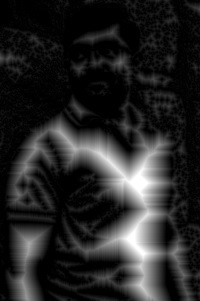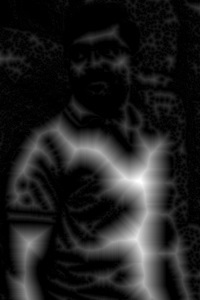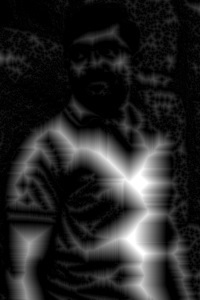# OpenCV - Distance Transformation

The distance transform operator generally takes binary images as inputs. In this operation, the gray level intensities of the points inside the foreground regions are changed to distance their respective distances from the closest 0 value (boundary).

You can apply distance transform in OpenCV using the method distanceTransform(). Following is the syntax of this method.

```distanceTransform(src, dst, distanceType, maskSize)
```

This method accepts the following parameters −

• src − An object of the class Mat representing the source (input) image.

• dst − An object of the class Mat representing the destination (output) image.

• distanceType − A variable of the type integer representing the type of the distance transformation to be applied.

• maskSize − A variable of integer type representing the mask size to be used.

## Example

The following program demonstrates how to perform distance transformation operation on a given image.

```import org.opencv.core.Core;
import org.opencv.core.Mat;
import org.opencv.imgcodecs.Imgcodecs;
import org.opencv.imgproc.Imgproc;

public class DistanceTransform {
public static void main(String args[]) {

// Reading the Image from the file and storing it in to a Matrix object
String file ="E:/OpenCV/chap19/input.jpg";

// Creating an empty matrix to store the results
Mat dst = new Mat();
Mat binary = new Mat();

// Converting the grayscale image to binary image
Imgproc.threshold(src, binary, 100, 255, Imgproc.THRESH_BINARY);

// Applying distance transform
Imgproc.distanceTransform(mat, dst, Imgproc.DIST_C, 3);

// Writing the image
Imgcodecs.imwrite("E:/OpenCV/chap19/distnceTransform.jpg", dst);

System.out.println("Image Processed");
}
}
```

Assume that following is the input image input.jpg specified in the above program.## Output

On executing the program, you will get the following output −

```Image Processed
```

If you open the specified path, you can observe the output image as follows −## Types of Distance Transform Operations

In addition to the distance operation type DIST_C demonstrated in the previous example, OpenCV caters various other types of distance transform operations. All these types are represented by predefined static fields (fixed values) of Imgproc class.

You can choose the type of the distance transform operation you need, by passing its respective predefined value to the parameter named distanceType of the distanceTransform() method.

```// Applying distance transform
Imgproc.distanceTransform(mat, dst, Imgproc.DIST_C, 3);
```

Following are the values representing various types of distanceTransform operations and their respective outputs.

Operation and Description Output
DIST_CDIST_L1DIST_L2DIST_LABEL_PIXELDIST_MASK_3